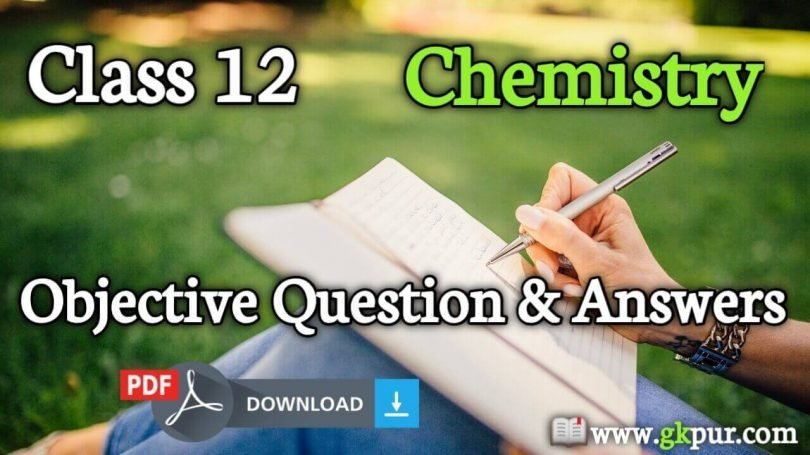# Class 12th Chemistry Objective Question And AnswerHi, friends on this page we are sharing the 12th Chemistry Objective Questions And Answers PDF download link. This Chemistry MCQ Question Answer is very useful for students studying. This can be vvi important Question For Board Exam 2021-22.

Class 12th Chemistry Objective Questions and Answers | Chemistry MCQ questions and answers | Chemistry objective question for class 12 |12th Chemistry Objective Questions | 12th board VVI question 2021-22.

## Class 12th Chemistry Objective Question And Answer

1. The number of particles in a simple cubic per unit cell is :

• One
• two
• three
• four
One

2. The number of particles in a face-centered cubic (fcc) per unit cell is

• one
• two
• three
• Four
Four

3. The number of particles in a body-centered cubic (bcc) per unit cell IS :

• one
• Two
• Three
• Four
Tw0

4. Graphite is an example of

• Ionic Solid
• Molecular Solid
• Covalent Solid
• Metallic Solid
Covalent Solid

5. Possible Co-ordination number of Cation of tetrahedral structural Arrangement is:

• 1
• 2
• 3
• 4
4

6. The molecularity of a reaction cannot be :

• Zero
• One
• Two
• Three
Zero

7. Cuprite is an ore of :

• Zn
• Cu
• Ag
• Fe
Cu

8. Calamine is an ore of :

• Cu
• Fe
• Al
• Zn
Zn

9. Bauxite is an ore of :

• Al
• Fe
• Cu
• Zn
Al

10. Sulfide ores are generally concentrated by :

• Gravity separation method
• Magnetic separation method
• Froth floatation process
• None of these
Froth floatation process

11. Haematite is an ore of :

• Al
• Fe
• Cu
• Zn
Fe

12. Unit Of rate constant for Second-order reaction, K is :

• s⁻¹
• mol⁻¹ L s⁻¹
• mol⁻² L² s⁻¹
• mol L⁻¹ s⁻¹
mol⁻¹ L s⁻¹

13. Unit Of rate constant for First-order reaction, K is :

• s⁻¹
• mol⁻¹ L s⁻¹
• mol⁻² L² s⁻¹
• mol L⁻¹ s⁻¹
s⁻¹

14. Unit Of rate constant for the third-order reaction, K is :

• s⁻¹
• mol⁻¹ L s⁻¹
• mol⁻² L² s⁻¹
• mol L⁻¹ s⁻¹
mol⁻² L² s⁻¹

15. When nitroethane is treated with Li Al H4 the product formed is :

• Methylamine
• Ethylamine
• Ethane
• Acetamide
Ethylamine

16. Aldehyde and ketone can be distinguished by :

• Tollen’s reagent
• LiAIH4
• HCN
• None of these
Tollen’s reagent

17. Among the halogen acids the one which is weakest is :

• HBr
• HI
• HF
• HCI
HF

18. Which of the following is an artificial sweetening agent :

• Aspartame
• Seconal
• Aspirin
• Sodium benzoate
Aspartame

19. Acid chloride is reduced to the aldehyde by :

• Wurtz reaction
• Fitting reaction
• Rosenmund reduction
• None of these
Rosenmund reduction

20. Tollen’s reagent is:

• A mixture of CuSO4 and NaOH
• A solution of copper sulfate, sodium citrate, and sodium carbonate
• Ammonical silver nitrate solution
• None of these
A solution of copper sulphate, sodium citrate and sodium carbonate

21. Phenol is less acidic than:

• Ethanol
• Methanol
• p-Nitrophenol
• p- methyl phenol
p-Nitrophenol

22. The hybridization of Xe F2 Is :

• sp³d²
• sp³d
• sp³
• sp³d³
sp³d

23. The hybridization of Xe F4 Is :

sp³d²

s³d
sp³
sp³d³

sp³d²

24. Which one of the following is not a protein:

• Nail
• DNA
• Hair
• Wool
DNA

25. Enzymes are:

• Carbohydrates
• Vitamins
• Proteins
• None of these
Proteins

26. Vitamin A is Called

• Retinol
• Ascorbic acid
• calciferol
• Tocopherol
Retinol

27. which of the following is not found in RNA

• Uracil
• Ammonium Acetate
• Guanine
Ammonium Acetate

28. PVC is a

• Condensation polymer
• copolymer
• None of these

29. Phenol is used as :

• Antiseptic
• Disinfectants
• Both a & b
• None
Both a & b

30. Antipyretics is a :

• Quinone
• Paracetamol
• Luminal
• None
Paracetamol

👉 12th Chemistry Objective Questions And Answers – PDF Download

•Bablu babu says:

Too oo useful

•Puja kumari says:

Puja kumari

•Vishal roy says:

Sir hamko pdf ke deara dounlod karna hai

error: Content is protected !!